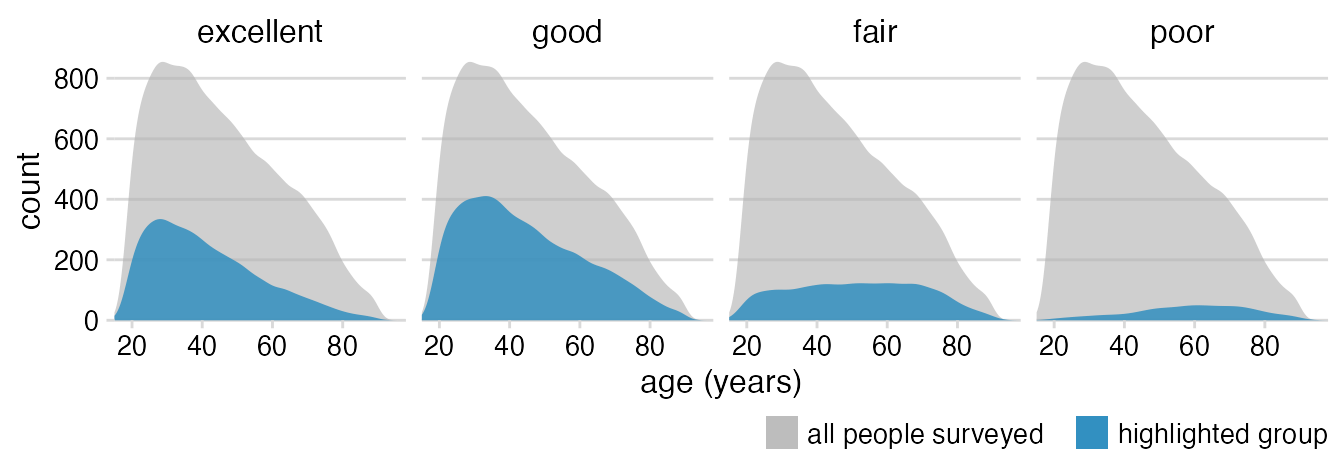For this example, we will use the following packages.

library(tidyverse)
library(cowplot)   # for theme_minimal_hgrid()

The dataset is provided as practicalgg::happy. Let’s look at it in table form and as basic density plots.

data_health <- practicalgg::happy %>%
select(age, health) %>%
na.omit() %>%
mutate(health = fct_rev(health)) # revert factor order

data_health
## # A tibble: 38,361 x 2
##      age health
##    <dbl> <fct>
##  1    23 good
##  2    70 fair
##  3    48 excellent
##  4    27 good
##  5    61 good
##  6    26 good
##  7    28 excellent
##  8    27 good
##  9    21 excellent
## 10    30 fair
## # … with 38,351 more rows
ggplot(data_health, aes(x = age, y = stat(count))) +
geom_density(fill = "lightblue") +
facet_wrap(~health, nrow = 1)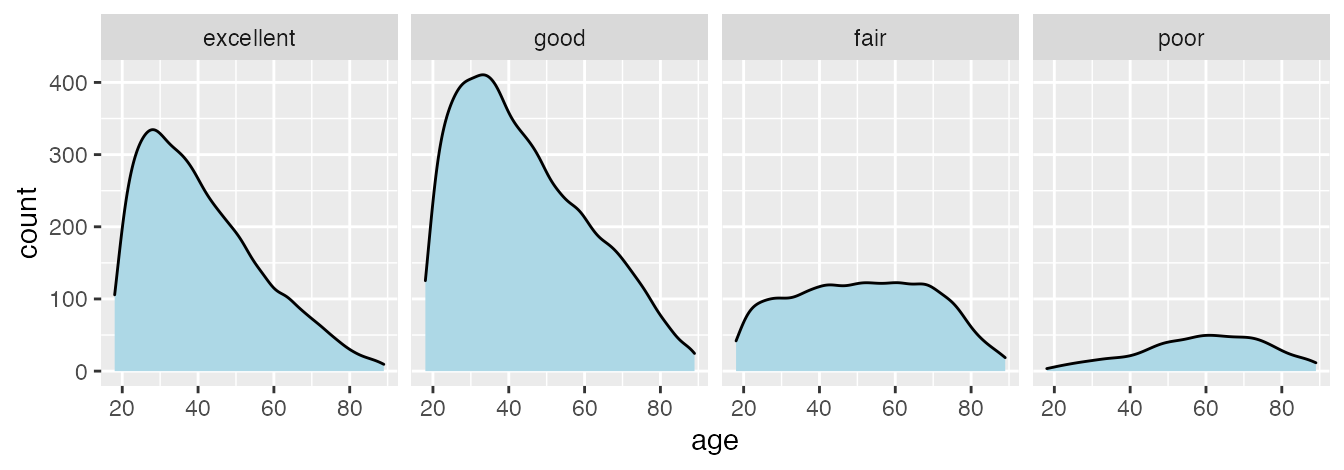Add the overall distribution as a background.

ggplot(data_health, aes(x = age, y = stat(count))) +
# we add a density layer that uses the data without the column
# by which we are faceting; this places the full dataset into
# each facet
geom_density(
data = select(data_health, -health),
aes(fill = "all people surveyed"),
) +
geom_density(aes(fill = "highlighted group")) +
facet_wrap(~health, nrow = 1)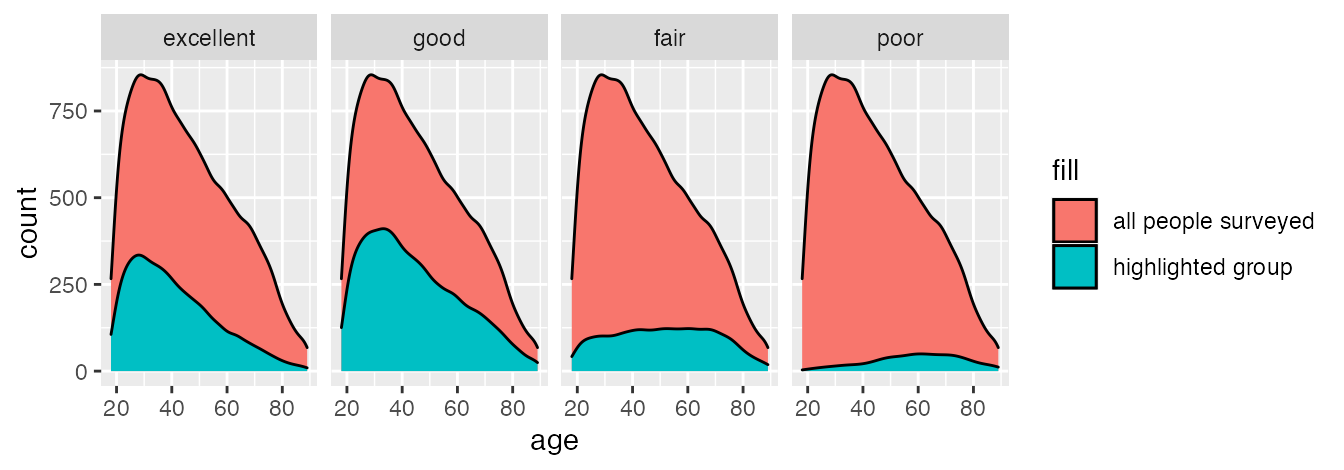Define the scales.

ggplot(data_health, aes(x = age, y = stat(count))) +
geom_density(
data = select(data_health, -health),
aes(fill = "all people surveyed"),
) +
geom_density(aes(fill = "highlighted group")) +
scale_x_continuous(
name = "age (years)",
limits = c(15, 98),
expand = c(0, 0)
) +
scale_y_continuous(
name = "count",
expand = c(0, 0)
) +
scale_fill_manual(
values = c("#b3b3b3a0", "#2b8cbed0"),
name = NULL,
guide = guide_legend(direction = "horizontal")
) +
facet_wrap(~health, nrow = 1)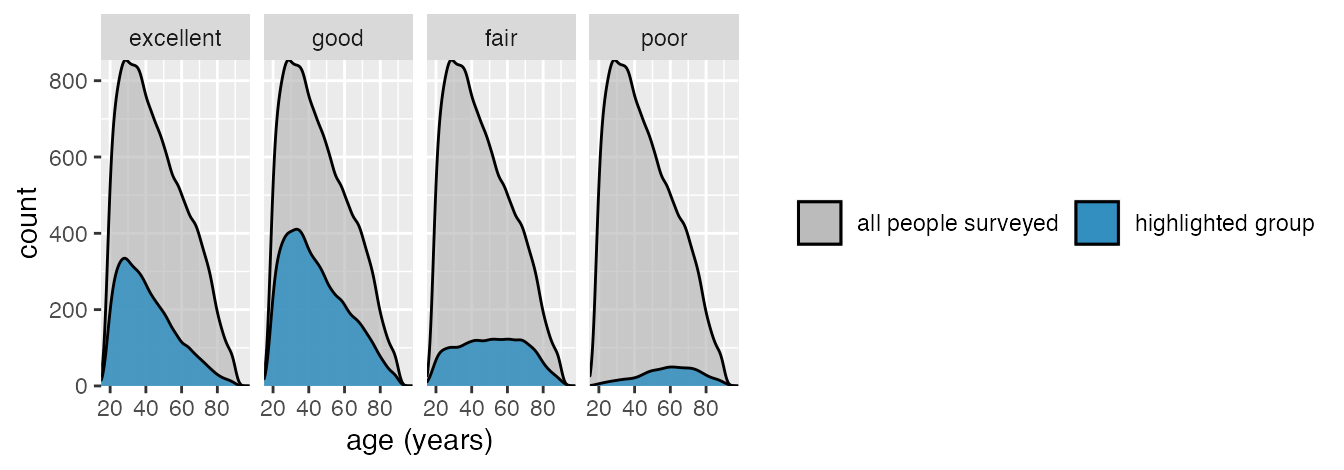Basic theme; move legend to bottom; remove outline around densities.

ggplot(data_health, aes(x = age, y = stat(count))) +
geom_density(
data = select(data_health, -health),
aes(fill = "all people surveyed"),
color = NA
) +
geom_density(aes(fill = "highlighted group"), color = NA) +
scale_x_continuous(
name = "age (years)",
limits = c(15, 98),
expand = c(0, 0)
) +
scale_y_continuous(
name = "count",
expand = c(0, 0)
) +
scale_fill_manual(
values = c("#b3b3b3a0", "#2b8cbed0"),
name = NULL,
guide = guide_legend(direction = "horizontal")
) +
facet_wrap(~health, nrow = 1) +
theme_minimal_hgrid(12) +
theme(
legend.position = "bottom",
legend.justification = "right"
)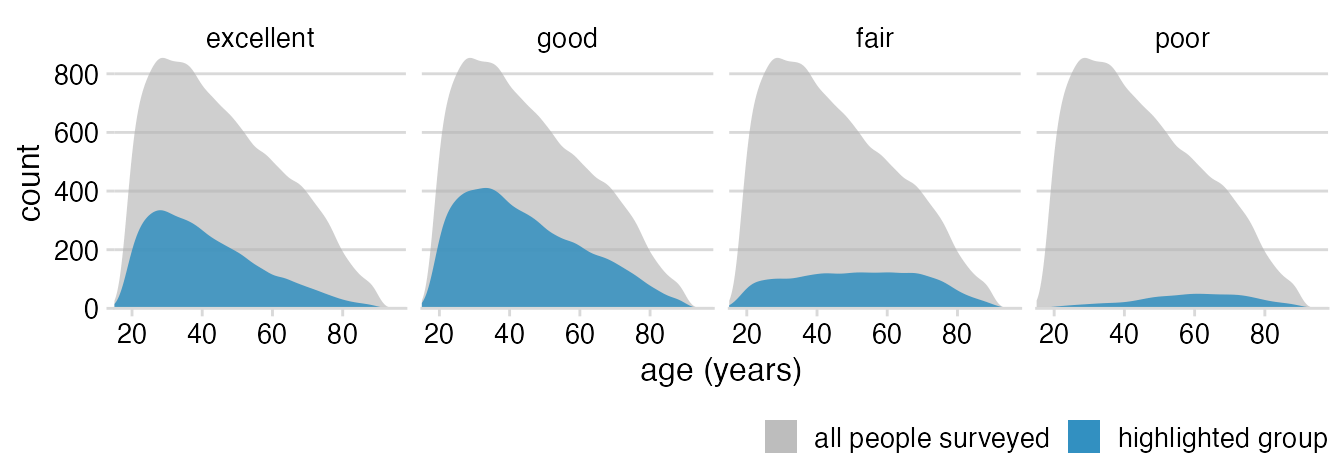Theme tweaks: Larger strip labels, move legend closer to plot, adjust horizontal legend spacing.

ggplot(data_health, aes(x = age, y = stat(count))) +
geom_density(
data = select(data_health, -health),
aes(fill = "all people surveyed"),
color = NA
) +
geom_density(aes(fill = "highlighted group"), color = NA) +
scale_x_continuous(
name = "age (years)",
limits = c(15, 98),
expand = c(0, 0)
) +
scale_y_continuous(
name = "count",
expand = c(0, 0)
) +
scale_fill_manual(
values = c("#b3b3b3a0", "#2b8cbed0"),
name = NULL,
guide = guide_legend(direction = "horizontal")
) +
facet_wrap(~health, nrow = 1) +
theme_minimal_hgrid(12) +
theme(
strip.text = element_text(size = 12, margin = margin(0, 0, 6, 0, "pt")),
legend.position = "bottom",
legend.justification = "right",
legend.margin = margin(6, 0, 1.5, 0, "pt"),
legend.spacing.x = grid::unit(3, "pt"),
legend.spacing.y = grid::unit(0, "pt"),
legend.box.spacing = grid::unit(0, "pt")
)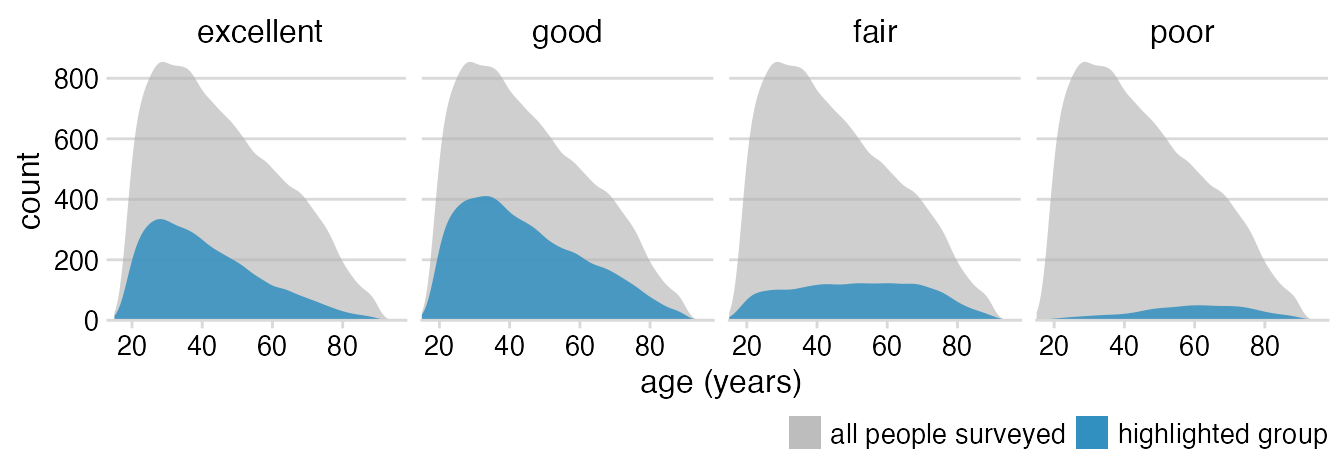Remove axis line, add spacing between legend items.

ggplot(data_health, aes(x = age, y = stat(count))) +
geom_density(
data = select(data_health, -health),
# a simple workaround to a limitation in ggplot2:
# add a few spaces at the end of the legend text
# to space out the legend items
aes(fill = "all people surveyed   "),
color = NA
) +
geom_density(aes(fill = "highlighted group"), color = NA) +
scale_x_continuous(
name = "age (years)",
limits = c(15, 98),
expand = c(0, 0)
) +
scale_y_continuous(
name = "count",
expand = c(0, 0)
) +
scale_fill_manual(
values = c("#b3b3b3a0", "#2b8cbed0"),
name = NULL,
guide = guide_legend(direction = "horizontal")
) +
facet_wrap(~health, nrow = 1) +
theme_minimal_hgrid(12) +
theme(
axis.line = element_blank(),
strip.text = element_text(size = 12, margin = margin(0, 0, 6, 0, "pt")),
legend.position = "bottom",
legend.justification = "right",
legend.margin = margin(6, 0, 1.5, 0, "pt"),
legend.spacing.x = grid::unit(3, "pt"),
legend.spacing.y = grid::unit(0, "pt"),
legend.box.spacing = grid::unit(0, "pt")
)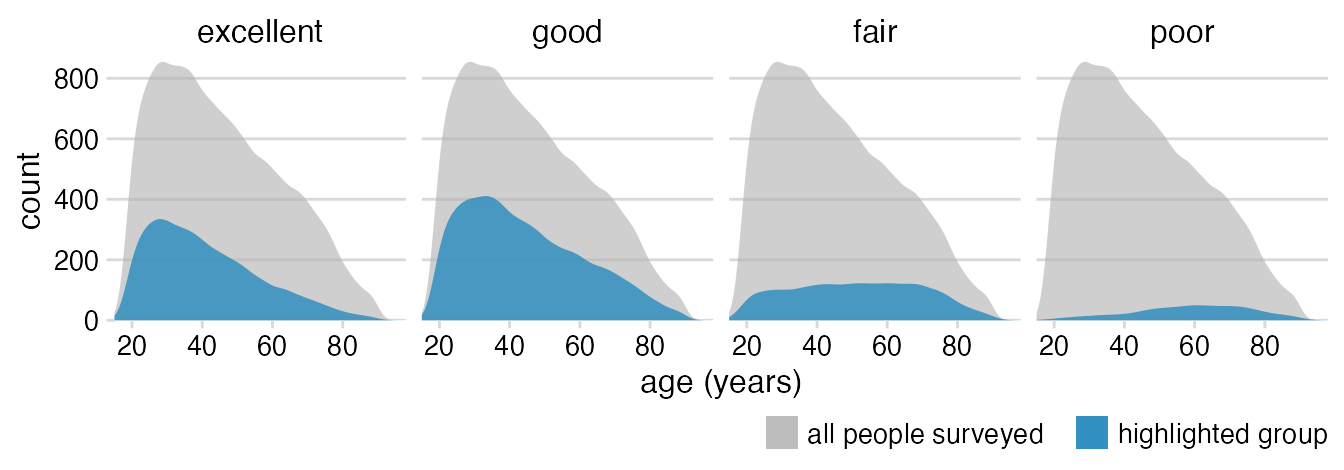Turn off clipping.

ggplot(data_health, aes(x = age, y = stat(count))) +
geom_density(
data = select(data_health, -health),
aes(fill = "all people surveyed   "),
color = NA
) +
geom_density(aes(fill = "highlighted group"), color = NA) +
scale_x_continuous(
name = "age (years)",
limits = c(15, 98),
expand = c(0, 0)
) +
scale_y_continuous(
name = "count",
expand = c(0, 0)
) +
scale_fill_manual(
values = c("#b3b3b3a0", "#2b8cbed0"),
name = NULL,
guide = guide_legend(direction = "horizontal")
) +
facet_wrap(~health, nrow = 1) +
coord_cartesian(clip = "off") +
theme_minimal_hgrid(12) +
theme(
axis.line = element_blank(),
strip.text = element_text(size = 12, margin = margin(0, 0, 6, 0, "pt")),
legend.position = "bottom",
legend.justification = "right",
legend.margin = margin(6, 0, 1.5, 0, "pt"),
legend.spacing.x = grid::unit(3, "pt"),
legend.spacing.y = grid::unit(0, "pt"),
legend.box.spacing = grid::unit(0, "pt")
)